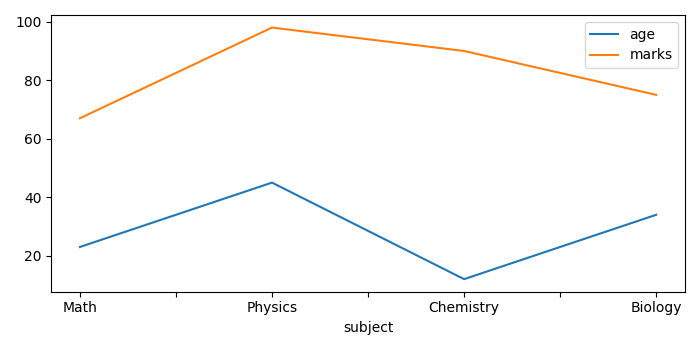# Plotting multiple dataframes using Pandas functionality

To plot multiple dataframes using Pandas functionality, we can take the following steps −

• Set the figure size and adjust the padding between and around the subplots.
• Create two Pandas dataframes, df1 and df2, of two-dimensional, size-mutable, potentially heterogeneous tabular data.
• Plot df1 and df2 using plot() method.
• To display the figure, use show() method.

## Example

import pandas as pd
from matplotlib import pyplot as plt

plt.rcParams["figure.figsize"] = [7.00, 3.50]
plt.rcParams["figure.autolayout"] = True

df1 = pd.DataFrame(
dict(
name=['John', 'James', 'Stephen', 'Kandy'],
age=[23, 45, 12, 34]
)
)

df2 = pd.DataFrame(
dict(
subject=['Math', 'Physics', 'Chemistry', 'Biology'],
marks=[67, 98, 90, 75]
)
)

ax = df1.plot(x='name', y='age')
df2.plot(ax=ax, x='subject', y='marks')

plt.show()

## Output

It will produce the following output